### Gordon W. Holtgrieve and Daniel E. Schindler. 2011. Marine-derived nutrients, bioturbation, and ecosystem metabolism: reconsidering the role of salmon in streams. Ecology 92:373–385.

Appendix B. Bayesian Metabolic Model description and example fits to data.

We used the Bayesian Metabolic Model (BaMM) to quantify metabolic rates and their uncertainness for the 10 diel sampling periods conducted in Pick Creek during the 2008 salmon season. A full description of the dynamic oxygen model and the Bayesian methods used to estimate metabolic rates can be found in Holtgrieve et al. (2010). Below is an abbreviated description of the methodology relevant to the current study.

For all 10 analyses, BaMM was supplied with water temperature and [O2] at 10 min intervals. When measured irradiance data were available for all time points being modeled (i.e., at 10 min intervals) it was supplied directly to the model as well. Otherwise we used modeled irradiance curves based on the geographic position and day of year, and scaled these curves to local conditions using available surface irradiance measurement (partial irradiance data were available for all dates). Oxygen isotope ratio data (δ18O-O2) were available for five of the 10 diel oxygen analyses (28–30 Jun, 14–15 Jul, 18–20 Aug, 29–31 Aug, 04–05 Sep).

Model description

The dissolved oxygen pool in an aquatic ecosystem is controlled by three simultaneous processes: (1) respiration (here defined as all oxygen consuming reactions within an ecosystem), (2) production of oxygen by photosynthesis (autotrophic production), and (3) reaeration or gas exchange with the atmosphere. When metabolic rates are large relative to the dissolved oxygen pool there are pronounced diel changes in both oxygen concentration ([O2]) and the oxygen 18O to 16O ratio (δ18O-O2). Oxygen concentrations build with increasing light intensity (irradiance) as photosynthesis releases O2 into the water. Respiration is a function primarily of temperature, and thus is relatively constant, resulting in a gradual draw down in dissolved oxygen during periods with low light intensity. Differences between daytime increases and nighttime declines in dissolved oxygen can therefore provide information on rates of photosynthesis and respiration, assuming reaeration can be adequately determined The additional measurement of the δ18O of dissolved oxygen provides a second, independent oxygen budget to quantify rates of biological processes (Quay et al. 1995, Venkiteswaran et al. 2007). Because the δ18O of local water is usually substantially depleted relative to atmospheric O2, the addition of O2 from photosynthesis lowers the δ18O-O2 while preferential uptake of 16O over 18O by respiration (fractionation) will enrich this pool.

Given a set of model parameters describing photosynthesis, respiration, reaeration, and initial conditions, BaMM generates trajectories of dissolved oxygen ([O2]) and δ18O-O2 through time. These trajectories are then compared to observed diel data, which, using Bayesian methods, allows for the selection of parameter values that do the best job of reconstructing the observed data. To generate dissolved oxygen trajectories, BaMM relies on a series of interrelated equations that describe, photosynthesis, respiration, and reaeration, and combines these processes to construct a mass balance of O2 and 18O-O2 concentration.

Model components

Photosynthesis

Photosynthesis was modeled as having a light saturation relationship for all but one of the diel sampling periods. Equation 1 calculates photosynthesis (P) as a function of irradiance (I) when light is saturating: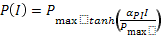(B.1)

where I is the instantaneous irradiance (µE s-1 m-2),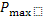is production of oxygen at light saturation, and αPI is the slope of the photosynthesis-irradiance relationship at low light conditions (Jassby and Platt 1976). Photosynthesis can also be modeled simple linear function of irradiance, if irradiance is assumed to be well below saturating conditions: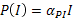(B.2)

The assumption of light-saturation can be tested using the negative log-likelihood of the model fits and Akaike’s Information Criterion (Burnham and Anderson 2002, Holtgrieve et al. 2010). For the 13–14 Aug 2008 diel sampling period, BaMM was unable to find constrained solutions for bothand αPI and therefore photosynthesis was modeled as a linear function.

Respiration

Respiration (R) was modeled as a temperature dependent process as described by the van ‘t Hoff–Arrhenius equation (Venkiteswaran et al. 2007 and reference therein).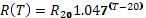(B.3)

T is the observed water temperature in °C. This model assumes all variation in R is a function of water temperature. In this construct, respiration includes all oxygen consuming reactions within the stream including heterotrophic respiration, chemical oxidation (i.e., nitrification), and photo-oxidation.

Gas exchange

The rate of diffusion of O2 across the air-water interface is controlled by the air-water O2 concentration gradient and the gas transfer velocity, k, with units of m h-1. The magnitude of the gas transfer velocity is a function of physical conditions within the aquatic system including surface turbulence, water viscosity, and the solubility of O2. Surface turbulence in streams is controlled by factors relating to discharge such as water depth and velocity (O'Connor and Dobbins 1958). Water viscosity and solubility are a function of water temperature (Wanninkhof 1992). BaMM includes k at 20°C for freshwater (k20) as an estimated parameter, which is internally adjusted for changing water temperature for each modeled time point using Schmidt numbers (Sc), which combine the effects of viscosity and solubility on gas transfer (from Wanninkhof 1992).

Mass balance equations

The equation describing the time rate of change of dissolved oxygen within a well mixed body of water is: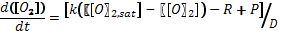(B.4)

where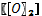is the dissolved oxygen concentration in mg m-3,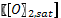is the dissolved oxygen concentration at atmospheric equilibrium, R is the instantaneous respiration rate, and P is the instantaneous rate of photosynthesis (R and P are in units of mg O2 m-2 h-1). D is the depth of the stream in meters. The first term in the numerator of equation 6 describes the net effect of gas exchange with atmosphere, which is the gas transfer velocity (k) times the O2 concentration gradient. The amount of dissolved O2 at equilibrium with the atmosphere () is calculated for a given water temperature using the equations from Weiss (1970).

The concentration of 18O as dissolved oxygen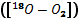is controlled by the same processes as [O2], but must be adjusted for process-specific fraction (described by the fractionation factor, α) and the isotopic ratios of the given pools. The equation incorporating these processes is: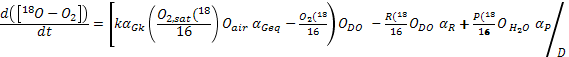(B.5)

where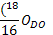is the isotopic ratio of dissolved oxygen,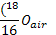is the isotopic ratio of atmospheric O2 (equal to 0.00205232), and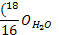is the measured isotopic ratio of oxygen in the water molecule at the time of sampling. αR, αP, αGk, and αGeq are the fractionation factors for respiration, photosynthesis, and the kinetic and equilibrium effects of gas exchange respectively (see Table 2 in Holtgrieve et al., 2010, for specific values).

Bayesian analysis

In BaMM, different sets of parameter combinations that describe the oxygen dynamic in the stream are considered as competing models and compared against each other as accurate descriptions of nature (Hilborn and Mangel 1997). Bayes Law applies, which defines the normalized posterior probability of the model given our observed data as: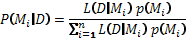(B.6)

where, L is the likelihood of observing the data (D) given the model (Mi) times any prior probabilities, p(Mi), describing prior information about one or more of the parameters. The combined likelihood of the data is the product of the individual likelihoods for observed [O2] data and, if available, δ18O-O2, and calculated as: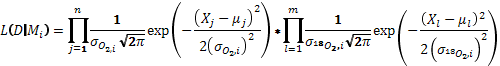(B.7)

where j = 1, …, n are the individual [O2] data points and l = 1 , …, m are the δ18O-O2 data points. Xj,l is the observed value for either [O2] or δ18O-O2, while µj,l is the predicted value. σO2 and σ18O2 are the standard errors around [O2] and δ18O-O2 values. The total likelihood of the model (L) was weighted such that each oxygen budget contributed equally to parameter estimates. Prior information (p(Mi)) about k20, σO2, and σ18O2 was specified as uniform distributions (a range of equally probable values). When including δ18O-O2 data , the fractionation of O2 from respiration (αR) was fixed at 1.000 based on previous analyses from Holtgrieve et al. (2010), while the δ18O-H2O was set at the measured value and assumed to be known without any uncertainty.

In contrast to optimization methods that seek to find the single combination of parameters that provides the absolute best fit to the data, Bayesian methods seek to find the range of parameter values that give reasonable fits to the data and express those ranges in terms of probability. BaMM identifies parameter combinations resulting in high posterior probabilities of the model given the data (). This is achieved though implementation of a Markov Chain Monte Carlo (MCMC) algorithm which generates a random-walk through the parameter space sampling values of each parameter in proportion to the total likelihood. With a sufficient number of steps, the MCMC chain will converge to the Bayesian posterior distribution for each of the estimated parameters in the model. To ensure the full posterior distribution has been explored, the MCMC chain is “thinned” by saving draws at a sufficient interval such that successive draws are uncorrelated (typically every 1000 steps in the chain).

Model implementation

MCMC chains were from one to five million iterations and thinned to 1000 saved posterior draws. Autocorrelation among saved draws was less than 0.05 for all parameters in the model, indicating the MCMC chains had sufficiently explored the posterior space (Branch et al. 2007). With the exception of the 13–14 August 2008 period, all diel data were best fit using a light-saturating model of photosynthesis compared to a linear model as determined by comparing the Akaike’s Information Criterion among the competing production models (Burnham and Anderson 2002).

Example fits to observed data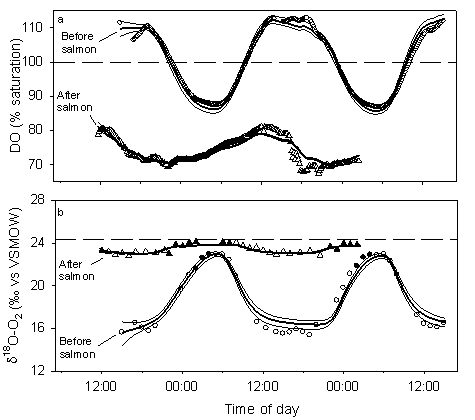FIG. B1. Examples of measured and model-predicted diel changes of dissolved O2 pools in Pick Creek before and after salmon entered the ecosystem. Before salmon samples are from 28–30 Jun 2008 while during salmon samples are from 18–20 Aug 2008. Thick lines are the mean predicted O2 and δ18O-O2; thin lines are the 2.5% and 97.5% credible limits. Filled symbols indicate samples taken at night. Horizontal dashed lines indicate conditions at equilibrium with the atmosphere. Oxygen isotope data are referenced to Vienna Standard Mean Ocean Water (VSMOW)

LITERATURE CITED

Branch, T. A., E. M. N. Abubaker, S. Mkango, and D. S. Butterworth. 2007. Separating southern blue whale subspecies based on length frequencies of sexually mature females. Marine Mammal Science 23:803–833.

Burnham, K. P. and D. R. Anderson. 2002. Model selection and multimodel inference: A practical information-theoretic approach. 2nd edition. Springer-Verlag, New York, New York.

Hilborn, R. and M. Mangel. 1997. The ecological detective: Confronting models with data. Princeton University Press, Princeton, New Jersey.

Holtgrieve, G. W., D. E. Schindler, T. A. Branch, and Z. T. A'Mar. 2010. Simultaneous quantification of aquatic ecosystem metabolism and re-aeration using a Bayesian statistical model of oxygen dynamics. Limnology and Oceanography 55:1047–1063.

Jassby, A. D. and T. Platt. 1976. Mathematical formulation of the relationship between photosynthesis and light for phytoplankton. Limnology and Oceanography 21:540–547.

O'Connor, D. J. and W. E. Dobbins. 1958. Mechanism of reaeration in natural streams. Transactions of the American Society of Civil Engineers 123:641–684.

Odum, H. T. 1956. Primary production in flowing waters. Limnology and Oceanography 1:102–117.

Quay, P. D., D. O. Wilbur, J. E. Richey, A. H. Devol, R. Benner, and B. R. Forsberg. 1995. The O-18/O-16 of dissolved-oxygen in rivers and lakes in the Amazon basin: Determining the ratio of respiration to photosynthesis rates in freshwaters. Limnology and Oceanography 40:718–729.

Venkiteswaran, J. J., L. I. Wassenaar, and S. L. Schiff. 2007. Dynamics of dissolved oxygen isotopic ratios: A transient model to quantify primary production, community respiration, and air-water exchange in aquatic ecosystems. Oecologia 153:385–398.

Wanninkhof, R. 1992. Relationship between wind-speed and gas exchange over the ocean. Journal of Geophysical Research-Oceans 97:7373–7382.

Weiss, R. F. 1970. Solubility of nitrogen, oxygen and argon in water and seawater. Deep-Sea Research 17:721–735.

[Back to E092-033]